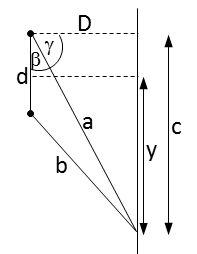# Two identical loudspeakers are 2 m apart. A person stands 4.6 m from one speaker and 3.4 m from...

## Question:

Two identical loudspeakers are 2 m apart. A person stands 4.6 m from one speaker and 3.4 m from the other. What is the lowest frequency at which destructive interference will occur at this point?

## Sound interference

Although Young's double slit experiment was performed with light, interference is a phenomenon typical of all waves. Therefore, if two point sources of sound are separated from each other, they will behave like Young's two slits. Therefore, the position of the interference minimums will be determined by the equation:

{eq}d\sin\theta=\left (m+\frac{1}{2} \right )\lambda {/eq}

In this case it is fulfilled that: {eq}\lambda=\frac{340\,\mathrm{m/s}}{f} {/eq}

where 340 m/s is the speed of propagation of sound in the air.

First we will calculate the distance that separates the person from the center of the interference pattern and then the distance that separates the speakers from the plane where the interference pattern is being heard. The figure shows the known quantities and those that will be calculated.{eq}b^2=d^2+a^2-2ad\cos\beta\Rightarrow \beta=\arccos\left ( \frac{d^2+a^2-b^2}{2ad} \right )=\mathrm{arccos\left ( \frac{(2\,m)^2+(4.6\,m)^2-(3.4\,m)^2}{2(4.6\,m)(2\,m)} \right )}=42.3^\circ\\ \gamma=90^\circ-42.3^\circ=47.7^\circ\\ \left.\begin{matrix} \sin\gamma=\frac{c}{a}\\ y=c-\frac{d}{2} \end{matrix}\right\}\Rightarrow y=a\sin\gamma-\frac{d}{2}=\mathrm{(4.6\,m)sin47.7^\circ-\frac{2\,m}{2}=2.4\,m}\\ \cos\gamma=\frac{D}{a}\Rightarrow D=a\cos\gamma=\mathrm{(4.6\,m)cos47.7^\circ=3.1\,m} {/eq}

Using now the equation corresponding to the interference minimums, we can find the minimum frequency at which destructive interference occurs:

{eq}\left.\begin{matrix} d\sin\theta=\left (m+\frac{1}{2} \right )\frac{340\,\mathrm{m/s}}{f}\\ \sin\theta=\frac{1}{\sqrt{(D/y)^2+1}}\\ m=0 \end{matrix}\right\}\Rightarrow f=\frac{170\,\mathrm{m/s}}{d}\sqrt{\left ( \frac{D}{y} \right )^2+1}=\mathrm{\frac{170\,m/s}{2\,m}\sqrt{\left ( \frac{3.1\,m}{2.4\,m} \right )^2+1}=0.15\,kHz} {/eq}